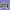Australia's Leading Digital Marketing Experts. T. 1300 235 433  |  Aggregation Enquires Welcome

# Convert Decimal Latitude & Longitude to Degrees, Minutes and Second

In a number of the applications we've built in the past we've had a need to manufacture traditional latitude and longitude coordinates from the decimal value. The following functions will convert decimal coordinates to long-hand, and we've also provided a function that'll perform the reverse operation.

### Understanding Latitude and Longitude

If you cut up the Earth into 360 'pieces' from around the center, each piece would represent a degree of longitude (360 degrees in total). If each of those 360 pieces were further divided up into 60 pieces, we'd be left with 21,600 pieces in total... or 21,600 minutes. Each minute of longitude is a nautical mile (meaning that the circumference of the Earth is 21,600 nautical miles). Further divide each minute of longitude into 60 pieces and you have 60 seconds of distance.Lines of Latitude and Longitude. Source: Wikipedia

A vertical line called the Prime Meridian, at 0 degrees longitude, and its twin line of longitude, opposite the Prime Meridian at 180 longitude, divides the earth into the Eastern (0 to 180°) and Western Hemispheres (0 to -180°). The Equator cuts the Earth into two halves (0 to 90° and 0 to -90°), the upper being the Northern hemisphere and the lower the Southern.

The purpose of this ramble was to illustrate the base of 60 so the PHP function below makes more sense.

### Why Decimal Coordinates are Used

Decimal coordinates are more common that long-hand these days because they're more machine readable (in URLs etc.) and more easily stored in a database. As such, they're used in numerous geographic information systems (GIS) web and map applications, such as Google Maps.

Using decimal coordinates, it's very easy to express highly accurate coordinates. For example, 5 decimal places provides accuracy to around a meter (around 3.3 feet) while 6 decimal places gives accuracy to around 10 centimetres (around 3 inches).

### Degrees, Minutes and Seconds

Using degrees, minutes and seconds in long-hand is more user friendly. It makes a position more immediately apparent without any calculation, and their use is the only means of plotting a position on a physical chart.

A DMS value is converted to decimal degrees using the formula:

DD = D + M/60 + S/3600

To calculate degrees, minutes and seconds to decimals, the following formula can be used:

D = trunc(D_(dec))

M = trunc((D_(dec) times 60) mod 60)

S = (|D_(dec)| times 3600) mod 60

In the world of PHP, these equations translate to the functions provided below.

### PHP Functions

The following function, provided with a decimal value, will return an array of degrees, minutes and seconds.

1
<?php
2
/*
3
Convert Decimal Latitude & Longitude to Degrees, Minutes and Second
4
http://www.beliefmedia.com/convert-decimal-latitude-longitude
5
*/
6

7
function beliefmedia_dec_dms($dec) { 8 9$vars = explode(&quot;.&quot;, $dec); 10$deg = $vars; 11$tempma = '0.' . $vars; 12 13$tempma = $tempma * 3600; 14$min = floor($tempma / 60); 15$sec = $tempma - ($min * 60);
16

17
return array('deg' => $deg, 'min' =>$min, 'sec' => $sec); 18 } 19 ?> Usage Consider the geographic coordinates of Sydney (-33.86785, 151.20732). Function usage (to return the latitude position) is as follows: 1 /* Print in pre tags */ 2 print_r(beliefmedia_dec_dms('-33.86785')); The result: 1 Array 2 ( 3 [deg] => -33 4 [min] => 52 5 [sec] => 4.26 6 ) Note that the degrees is -33, meaning South. If our input was 33.86785 it would have returned a positive number representing the neighboring coordinate in the Northern Hemisphere. To return a full location in with the N, S, E or W represented, we can hack together another function that uses PHP's strpos()function to determine if a "-" sign is present. If so, we'll prefix the output appropriately (with html entities for degrees, minutes and seconds. Decimal Latitude and Longitude to Degrees, Minutes and Seconds 1 <?php 2 /* 3 Convert Decimal Latitude & Longitude to Degrees, Minutes and Second 4 http://www.beliefmedia.com/convert-decimal-latitude-longitude 5 Requires: beliefmedia_dec_dms(); 6 */ 7 8 function beliefmedia_lat_lng($lat, $lng) { 9 10$latpos = (strpos($lat, '-') !== false) ? 'S' : 'N'; 11$lat = beliefmedia_dec_dms($lat); 12 13$lngpos = (strpos($lng, '-') !== false) ? 'W' : 'E'; 14$lng = beliefmedia_dec_dms($lng); 15 16 return$latpos . abs($lat['deg']) . '&deg;' .$lat['min'] . '&apos;' . $lat['sec'] . '&quot ' .$lngpos . abs($lng['deg']) . '&deg;' .$lng['min'] . '&apos;' . $lng['sec'] . '&quot'; 17 } Usage is as follows: 1 /* Usage */ 2 echo beliefmedia_lat_lng($lat = '-33.86785', $lng = '151.20732'); Our example will return the following: S33°52'4.26" E151°12'26.352" ### Converting Degrees, Minutes and Seconds to Decimal The following function can be used to generate a decimal figure for degrees, minutes and seconds. In our example we'll use the longitude for Sydney. 1 <?php 2 /* 3 Converting Degrees, Minutes and Seconds to Decimal 4 http://www.beliefmedia.com/convert-decimal-latitude-longitude 5 */ 6 7 function beliefmedia_dms_dec($deg, $min,$sec, $round = 0) { 8$dec = $deg + ((($min * 60) + ($sec)) / 3600); 9 if ($round != 0) $dec = round($dec, $round); 10 return$dec;
11
}
12

13
/* Usage */
14
echo beliefmedia_dms_dec('151', '12', '26.35');

The function will return 151.207319444. Optionally, you can provide a fourth argument to truncate and roundthe returned value. For example, echo beliefmedia_dms_dec('151', '12', '26.35', '4'); will return 151.2073.

Title: Convert Decimal Lat & Long to Degrees, Minutes and Second
Description: Convert Decimal Latitude & Longitude to Degrees, Minutes and Second (and vice versa).

Download our 650-page guide on Finance Marketing. We'll show you exactly how we generate Billions in volume for our clients.AUS Eastern Standard Time (Virginia)

Want to have a chat?